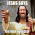## Monday, December 29, 2014

### NFL Picks Contest- Week 17 Results and Standings

FYI- As we enter the playoffs, ALL games will be eligible for the contest, even those played on Saturday.

Here are the Week 17 results and standings in the NFL Picks Contest. cokeboy99 extends his lead.

Here are the current standings;

cokeboy99- 27-(8)+21+34+16+13+20+38+16+51+43+13+32+16+20+39+24 = 415 pts.
OneAndDone- 27+17+17+34+41+5+31+38+16+24+17+30+32+16+18+27+20 = 410 pts.
MOJO-  27+17+17+29+16 +24+32+37+16+34+17+30+32+16+18+12+20 = 394 pts.
Music City Momma- 13+17+21+29+16-(7)+32+38-(3)+31+17+30+32+17+17+39+43 = 382 pts.
Vegas Vic- 27+17+21+29+16+5+32-(3)+20+31+17+29+32+16+17+39+24 = 369 pts.

Captain Crunch- 27+17+17+29+16+5+35+38+24+24-(17)+30+32+34+17+11+20 = 359 pts.
lightning36- 27+17+17+29+16+24+31+24+20+34-(15)+30+32-(31)+18+27+43 = 343 pts.
TwoMinuteWarning- (3)+17+37+29+16-(7)+35+37+20+24-(17)+19+32+17+18+39+24 = 337 pts.
Grange95- (3)+17+21+29+16+24+11+20+16+0+43+13+62+34-(8)+16+20 = 331 pts.
mrben09- 27+17+17+29+16+24+31+37-3+24-(15)+30+32+16+18+11+16 = 327 pts.

ggrouchie- 27+17+17+34+41+13+20-(3)+24+24-(15)+30+32+16+18-(2)+16 = 309 pts.
Neophyte- 27+17-(19)+0+41+24+21+0+24+24+17+14+32+24+28+0+0 = 274 pts.
Coach- 27+17+21+29+20+24+20-(3)+16-(7)-(20)+14+11+16+26+11+20 = 242 pts.

Week 17 scores

MOJO- Baltimore (W 20-10)= 20 pts.
Vegas Vic- Seattle (W 20-6)= 24 pts.
Captain Crunch- Baltimore (W 20-10)= 20 pts.
OneAndDone- Baltimore (W 20-10)= 20 pts.

mrben09- Houston (W 23-17)= 16 pts.
ggrouchie- Houston (W 23-17)= 16 pts.
Coach- Baltimore (W 20-10)= 20 pts.
lightning36- Denver (W 47-14)= 43 pts.

Neophyte- No Pick = 0 pts.
Music City Momma- Denver (W 47-14)= 43 pts.
TwoMinuteWarning- Seattle (W 20-6)= 24 pts.
Grange95- Green Bay (W 30-20) = 20 pts.
cokeboy99- Seattle (W 20-6)= 24 pts.

Thanks for playing.

Wild Card Playoff picks are due by Saturday at 11am (CST). All games are eligible.

Good luck!

#### 1 comment:

1.